# Test Case Study

## Brief Description of the Study Test Case

The geometry of the Sajben transonic converging-diverging diffuser and the flow characteristics have been obtained from the Alliance CFD Verification and Validation Archive-NASA website (http://www.grc.nasa.gov/WWW/wind/valid/archive.html). The diffuser geometry and the dimensions are given in fig.4. The flow starts subsonic with M=0.46 at the inlet of the diffuser, accelerates through the throat up to the supersonic region where a shock-wave is formed which abruptly decelerates the flow which is then subsonic in the remaining diverging part of the diffuser downstream of the shock-wave.

The test case geometry configuration has an entrance-to-throat ratio equal to 1.4 and an exit-to-throat ratio equal to 1.5. In the current study, two flow configurations were examined. Based on the Mach number that is reached in the diffuser, the configurations are named as the “weak” and the “strong” case. For the “weak” case, the flow accelerates up to Mach~1.25 and for the “strong” case up to Mach~1.35, is then decelerated by a weak or a strong shock-wave and leaves the diffuser at Mach=0.52 and Mach=0.65 respectively. The different flow development for the two cases is guided by the imposed static pressure at the outlet boundary of the Sajben diffuser and it is characterized by the ratio R of the outlet static pressure to the inlet total pressure of the flow. The ratio R takes the values of 0.82 and 0.72 for the “weak” and the “strong” Mach number cases respectively. For the weak case, the boundary layer stays attached, while it separates from the upper wall of the diffuser for the “strong” case leading to different flow development in the diverging part of the diffuser. The position of the “weak” and the “strong” shock-waves together with the corresponding recirculation region for the “strong” Mach number case are indicated in fig.4.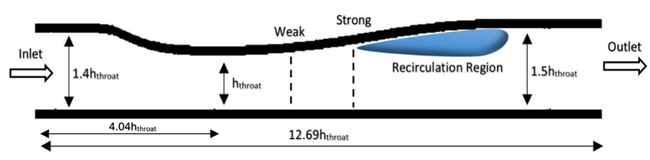Figure 4: Geometry of the Sajben diffuser and schematic representation of the shock-waves position and the recirculation region

The two test case setups regarding all the flow boundary conditions and the general characteristics e.g. Mach number values, total pressure, total temperature, outlet boundary static pressure, are provided in table 2.

Table 2: Geometry, boundary conditions and flow characteristics for the two setups
Case Total pressure Total temperature Outflow static pressure Maximum Mach number R
1 ‑ Weak 135000 (Pa) 277.78 (K) 110661 (Pa) 1.27 0.82
2 ‑ Strong 97217 (Pa) 1.35 0.72
hthroat=44 (mm)

The principal measured quantities that are available for the validation of the various computational CFD codes, are basically mean averaged quantities of the pressure distributions along the walls of the diffuser and the velocity distributions at various stations after the shock-wave. Unfortunately, there are no experimental data regarding the turbulent quantities in order to have a more qualitative assessment of the behavior of the adopted turbulence models.

## Test Case Experiments

### Experimental setup

The transonic diffuser experimental studies were conducted at McDonell Douglas research laboratories, St. Louis, Missouri, USA. Regarding the wind tunnel operation a brief description is given below based on Bogar et al. (1982). Dry, filtered air is supplied to the test section from a 51cm diameter plenum chamber which provides highly uniform low- turbulence air flow. The flow at the exit of the test section is ventilated to the atmosphere in order to provide a constant pressure boundary condition. The supplied air temperature variations provided a Reynolds number within the range of 6.4 – 8.4 × 105, based on diffuser throat height and conditions.

A characteristic sketch of the converging-diverging experimental test-section in which the measurements were carried out is shown in fig.5. The sidewalls of the experimental test section were made of 25.4mm thick schlieren quality glass, for optical diagnostic methods to be more easily applied. The diffuser configuration had an entrance- to-throat area ratio of 1.4, an exit-to-throat area ratio equal to 1.5 and the width-to-throat ratio of the area at the throat was 4.03. In order to have minimum reflectivity, all the optical surfaces were covered with dielectric coatings.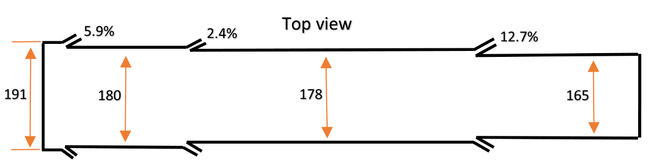Figure 5: Side and top sketch view of the experimental test section. Percentages indicate area decrease at slots. Slots sizes are enlarged. Dimensions are in mm. (The sketch and the comments were reproduced from Mohler (2005))

During the experiments it was observed that for maximum Mach numbers less than 1.27, the flow was fully attached. In cases where the Mach number exceeded the above limiting value, shock induced separation was observed in the diverging part of the diffuser. Two test cases were examined in the measurements, one having a “weak” and the other a “strong” Mach number that it is reached after the diffuser throat. The first one has Mach~1.25 and attached flow on both walls and the second one has Mach~1.35 resulting in shock induced boundary layer separation on the upper wall and a fully attached flow on the bottom wall. The boundary layer was tripped in the inlet at the top wall of the diffuser as indicated in fig.5. The top wall boundary layer at the throat was turbulent for all cases and the sidewall and bottom boundary layers were expected to be laminar up to the shock- wave. The experimental setup configuration provided highly uniform flow with low turbulence.

### Velocity and pressure measurements

For the measurements of the streamwise velocity the Laser Doppler Velocimetry (LDV) technique was used. The laser light source was a 4-W argon-ion laser which was operated at 514.5nm. The selected stations were located after the shock-wave which is formed just after the diffuser throat. The optical axis of the laser beam was normal to the diffuser sidewalls. The probe volume that was generated had a streamwise resolution of 0.46mm and a fringe spacing of 14μm. The velocity sampling ranged from approximately 500Hz near the walls up to more than 1.5kHz in the core flow. The mean velocity distributions were obtained by simple averaging the measured data. The measurement stations were aligned to positions after the shock-wave (positions selected for comparison with calculations are given in fig.9). For the “weak” Mach number case, 13 stations were selected and for the “strong” Mach number case 11 since, for the strong case the shock- wave was closer to the exit and the available space towards the exit of the diffuser was limited. The vertical measurement positions from the bottom up to the top wall were 29 for all axial stations, lying on the symmetry plane of the diffuser. The vertical spacing of the measurement positions was smaller near the walls and larger near the main core of the flow. The relative positions between the vertical measurement stations had an accuracy of 0.08mm. Regarding the mean static pressure distribution measurements, more than 100 pressure taps were placed on the bottom and top wall of the diffuser. Finally, for the visualization of the time-dependent shock location, a line-scan television-type camera combined with a shadowgraph optical system was used.

The measured flow development is shown in figs.6 and 7. Figure 6 presents characteristic spark schlieren photographs, Bogar et. al. (1982), while fig.7 presents the measured mean axial velocity for the “weak” and the “strong” Mach number cases, where the differences between the sub-sonic boundary layers between the two examined cases can be observed. The schlieren pictures of the flow field were made by capturing 5000 frames/sec and the flow field view for the photographs extended from X/hthroat= -1 to 8. For the “weak” Mach number case no recirculation is present and both boundary layers on top and bottom walls are attached being relatively thin. Furthermore, an inviscid region of constant total pressure is present in the core flow. On the other hand for the “weak” Mach number case, there is a separation observed on the top wall which rapidly thickens while the top and bottom boundary layers merge before the diffuser exit. The recirculation region is denoted by the shaded area in fig.7.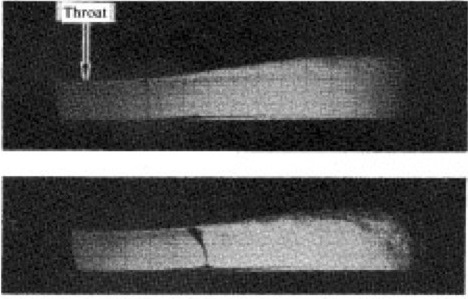Figure 6: Spark schlieren photographs of attached and separated flow. Top: “weak” Mach number case. Bottom: “strong” Mach number case. "From Bogar et al. (1982), doi: 10.2514/3.8234; reprinted by permission of the American Institute of Aeronautics and Astronautics, Inc."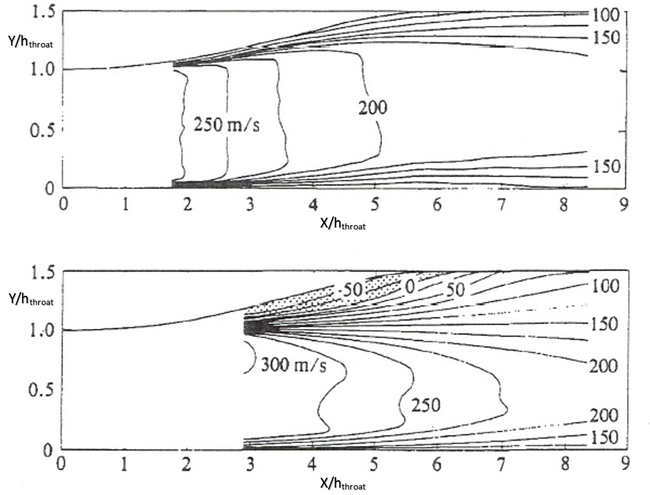Figure 7: Measured Velocity contours. Top: “weak” Mach number case. Bottom: “strong” Mach number case. "From Salmon et al. (1982), doi: 10.2514/3.8311; reprinted by permission of the American Institute of Aeronautics and Astronautics, Inc."

### Two-dimensionality of the flow

The boundary layer growth was limited by three sets of suction slots placed on the side and bottom walls, fig.5. More specifically, two slots upstream of the measurement area, where the flow was choked, and one downstream where the flow was nearly choked. This configuration obtained good approximation of a two-dimensional flow since the suction slots minimized the three-dimensional effects. The percentages that are shown in fig.5 are equal to the mass fraction that was removed from the diffuser, Bogar et al. (1982).

### General remarks on the experimental studies

• Apart from the measurements with no excitation at the outlet of the diffuser, measurements were also conducted for an excitation outlet frequency of 330Hz. The outlet excitation experiments were conducted in order to simulate the ramjet burner pressure oscillations. In the current work only the experimental cases with no outlet excitation were investigated.

## CFD Methods

For the CFD calculations of the current contribution, the “strong” and the “weak” Mach number cases of the Sajben transonic diffuser were investigated having a maximum Mach number ~1.35 and ~1.25 respectively, as measured by the experiments. The CFD code that was used for the study of the current UFR is an academic in-house flow solver that has been developed in the Laboratory of Fluid Mechanics and Turbomachinery (LFMT) at the Mechanical Engineering Department of the Aristotle University of Thessaloniki (AUTH). The code solves the Favre-averaged Navier-Stokes and energy equations governing compressible flow as will be discussed further below. The turbulence models for calculating the turbulent (Reynolds) stresses appearing in the averaged Navier-Stokes equations will be introduced first.

### Turbulence modelling

Three turbulence models were implemented that follow different turbulence modelling philosophies: the linear eddy-viscosity (LEVM) of Launder and Sharma (1974), the non-linear eddy-viscosity model (NLEVM) of Craft, Launder and Suga (1996) and the non- linear Reynolds-stress model (RSM) of Craft (1998). The models are implemented in the solver in their low-Reynolds number formulations, in order to integrate the transport equations up to the wall, resolve the flow quantities in all boundary layer regions and capture in a better manner the turbulent boundary layer/shock-wave interaction. The details of all the turbulence models with all the equations defining them are given in VY.

#### • The eddy-viscosity models (EVM)

The eddy-viscosity models are two-equation turbulence models that solve a transport equation for the turbulent kinetic energy k and a transport equation for the isotropic part of the turbulent dissipation ε*. For the modelling of the Reynolds-stresses, the LEVM adopts the linear Boussinesq approximation, while the NLEVM uses a non-linear cubic expression, which relates the Reynolds-stresses to products of strain and vorticity tensors. In order to fully describe the boundary layer behavior, appropriate near-wall damping functions for calculating the eddy-viscosity are used. Additionally, the NLEVM uses a strain- sensitized expression for calculating the Cμ coefficient of the eddy-viscosity. This equation enables the model to provide more accurate results in regions where turbulence is not in equilibrium.

#### • The Reynolds-stress model (RSM)

The adopted Reynolds-stress model solves six different transport equations, one for each Reynolds-stress component, and an additional transport equation for the isotropic part of the turbulent dissipation rate. For the modeling of the pressure-strain correlation terms, the model uses non-linear expressions relating the terms to turbulent stresses and velocity gradients.

In the current work, some minor modifications were applied to the original model of Craft (1998) in order to ensure convergence stability and, only for the “strong” Mach number case, some additional modifications were made in order to obtain physically consistent results. More specifically, in the original RSM the triple correlations needed for the calculation of the turbulent diffusion terms of the Reynolds-stresses are derived from a simplification of transport equations for these correlations. The convection and diffusion terms of the triple correlations transport equations are neglected, and a set of algebraic equations is solved for their calculation. This approach, which is quite sophisticated, incorporates more physical insight and should produce more realizable results, gave rise to instabilities during the calculations and often led to divergence of the solution. In order to overcome this numerical problem, two alternative expressions were adopted for the modeling of the triple correlations and hence the turbulent diffusion: the expression of Hanjalic and Launder (1972), which was also used in the work of Craft and Launder (1996), and the most widely used Generalized Gradient Diffusion Hypothesis (GGDH) of Daly and Harlow (1970). Both expressions gave similar results for the “weak” and the “strong” shock-wave cases. Hence, the final calculations were performed using the GGDH approach which was found to be more stable during the numerical calculations.

Some additional minor modifications were applied to the coefficient of the “fast” term component of the pressure-strain correlation model. The “fast” term describes the redistribution of the energy among the normal stresses and is responsible for the rapid return to isotropy mechanism of turbulence. This particular modification was found to have an impact only on the “strong” Mach number case. More specifically, without the adopted modification in the region of the “strong” shock-wave a deformation of the sharp shock-wave shape from the bottom wall up to the center flow of the duct was present, leading to a progressive decrease in the total predicted Mach number resulting in a solution which was lying in the subsonic flow regime. Although this particular defect was eliminated with the modification, the RSM was not able to predict the recirculation region formed right after the shock-wave in the diffuser diverging part and was hence not able to perform well for the “strong” Mach number case. The modified RSM was also found not to be able to reproduce very well the log-law in a simple boundary-layer flow on a flat plate (see VY). With these observations and although the study includes the RSM results for both “weak” and “strong” cases, it must be concluded that further research is necessary in order to investigate the effect of high Mach number compressibility on the pressure-strain correlation terms.

### Favre N-S equations, energy equation and heat fluxes

In order to take into consideration the mean density variations due to the transonic Mach number compressibility effects, the Favre-averaged formulation of the Navier-Stokes and energy equations were used. The total energy transport equation was incorporated into the solver and different expressions were used for the modelling of the turbulent heat fluxes, depending on the turbulence model adopted for calculating the Reynolds-stresses. For the EVMs, the eddy-diffusivity concept with the use of the turbulent Prandtl number was adopted, while for the RSM the GGDH was implemented. The same technique was also used by Batten et al. (1999) who modelled two- and three-dimensional compressible flows with the use of a non-linear RSM providing very good results in comparison to experimental data. Finally, for the correlation of the pressure-temperature-density variation the equation of state was used and Sutherland's law for the molecular viscosity was adopted in order to incorporate the viscosity changes due to the temperature variation. The equations are again all given in VY.

### Compressibility effects on turbulence

The transport equation for the turbulent dissipation refers only to the solenoidal dissipation of the turbulent kinetic energy. In order to take into account the compressible part of the dissipation, named dilatation dissipation, the proposal of Sarkar et al. (1989) was adopted. The dilatation dissipation is a function of the turbulent Mach number and the turbulent kinetic energy and it is proportional to the solenoidal dissipation rate. For the EVMs the dilatation-dissipation is incorporated into the transport equation of the turbulent kinetic energy by adding an extra source term to the turbulent transport equation. For the RSM an extra term is added to the Reynolds-stress dissipation rate tensor and as a result to the Reynolds-stress transport equations, but only for the normal Reynolds-stress components. As a final conclusion, the adopted compressibility corrections did not present any significant improvement of the final computational results, except for the LEVM and the “weak” Mach number case. The impact of the dilatation dissipation on the numerical results is presented in the Evaluation section of the current UFR.

### Numerical approach

The LFMT in-house academic CFD solver is a 3-D fully parallelized Navier-Stokes solver. The current 2D test case was calculated by implementing the pseudo-3D modelling approach in which one computational cell was used for the spanwise direction and by imposing symmetry boundary conditions on it. For the interpolation of the convection and diffusion terms, the second-order scheme of Zhu (1991) was adopted for all the transported variables in order to minimize the truncation error and to achieve a better accuracy in the final solution. The LFMT solver uses the SIMPLEC pressure-velocity coupling algorithm for incompressible flows. In order to expand the solver capabilities and account for the mean density variations due to the high speed of the flow, the technique that was developed by Lien and Leschziner (1994) was adopted. The main idea is to use an upwinding scheme in combination with a biasing function that was proposed by McGuirk and Page (1990) in order to weight the upstream influence of the mean density variations. The amount of upwind influence is guided by a “directional” Mach number, which is based on the contravariant velocity components of the computational cells. After using the density biasing expression for the continuity equation, and following the SIMPLEC algorithmic steps, a discretized transport equation for the pressure is formed, incorporating the compressibility effects as additional source terms. The total set of equations is closed with the use of the energy transport equation and the equation of state. As a result, a computational algorithm is built which is able to model flow fields from the incompressible subsonic regime up to the compressible transonic and supersonic flow regimes.

The computational grid used was a structured pseudo-3D H-type grid, having dimensions 105×81×2 nodes. The y+ was always kept less than 1 for at least 5 computational cells from the wall since all the turbulence models were based on low-Reynolds number formulations. A view of the computational grid is given in fig.8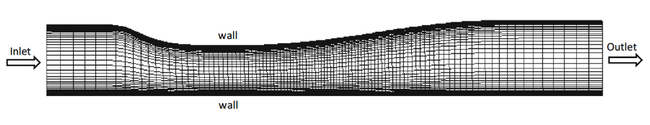Figure 8: Computational grid and domain

In order to diminish the unstable convergence behavior when the more advanced and complex models (NLEVM and RSM) were adopted by the solver, the calculations started with the LEVM, which was numerically stable, and then switched to the desired turbulence model after a certain number of iterations, usually defined after some trial and error attempts. The grid independency was also verified by performing calculations with a coarser and a finer grid for both “weak” and “strong” shock-wave cases. The results are presented in VY and the conclusion was that with the above grid the results are grid-independent.

Regarding the inlet values for the turbulent quantities, there is limited information found in the literature. In the present work, the inlet values for the turbulent quantities were obtained from the Alliance CFD Verification and Validation Archive-NASA website. However, it was observed that the inlet boundary conditions regarding the turbulent quantities had minor effect on the calculation of the velocities in the transonic diffuser after the shock-wave. The inlet boundary conditions that were used are summarized in table 3.

Table 3: Boundary conditions
Case Inlet total Pressure Inlet Total Temperature Outlet Static Pressure
1 ‑ Weak 135000 (Pa) 277.78 (K) 110661 (Pa)
2 ‑ Strong 97217 (Pa)
k = 1.74*10−3(m2/sec2) ε = 198 (m2/sec3)

During the iterative solution procedure, the inlet velocity was appropriately calculated at each iteration with respect to the fixed total inlet conditions, until it reached a constant value giving a Mach number equal to 0.46, for both “weak” and “strong” shock-wave cases.

Regarding the convergence of the numerical solution of the transport equations, a convergence criterion equal to 10-5 was chosen for the non-dimensional averaged values of pressure, energy and the velocity components. These non-dimensional averaged values have been computed by using the flux of the corresponding inlet values of the solved flow quantities. For the turbulent quantities, convergence was achieved when the dimensionless residuals had stabilized and had values at least lower by three orders of magnitude than the ones computed during the first iteration. Additionally, the maximum value of the Mach number was monitored and an additional convergence criterion was the reaching of the desired steady value for all the test cases. Further details regarding the CFD approach that was followed can be found in VY.

Contributed by: Z. Vlahostergios, K. Yakinthos — Dept. of Mechanical Engineering, Aristotle University of Thessaloniki, Greece